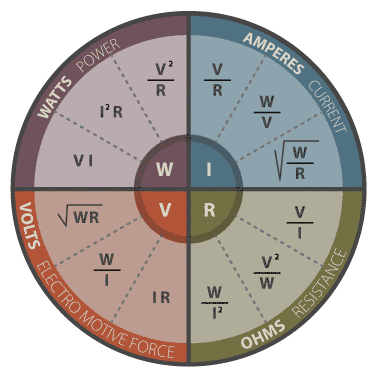# Ohm's Law

Enter any of the two values and then press "Calculate" to determine the other values.

• Volts (V)
• Amperes (A)
• Ohms (Ω)
• Watts  (W)

Ohm's Law describes the relationships that exist between voltage, current, and resistance. Quantities of these properties are expressed in volts (V); amperes, "amps" (A); and ohms (Ω) respectively. The product of voltage and current is the applied power and is expressed in watts (W).• E = IxR
• W = ExI